## Given problem

Suppose we have an array, we need to sort this array followed by increasing order.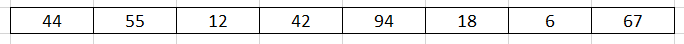In this article, we will use selection sort to deal with this problem.

## How selection sort works

The idea of the selection sort is that:

• at the step ith, we need to choose the mimimum element in the array `a[i], ..., a[n - 1]`.
• Then, iterate all element from `i + 1` to `n - 1`, compare `a[i]` and `a[j]` with `j = i + 1, ..., n`, and save the index of the minimum element in the `minIndex` variable.

• At the end of ith loop, we will exchange value between `a[i]` and `a[minIndex]`.

• Finally, we will have a sorted array.

Belows are some steps to describe the implementation of selection sort.

1. Original array2. At 0th position, select `a` as the minimum element of the array has range from 0 to `n - 1`.

• Iterate all elements from 1 to n - 1.

• Find the index = 6 is the minimum element.

• Swap `a` and `a`.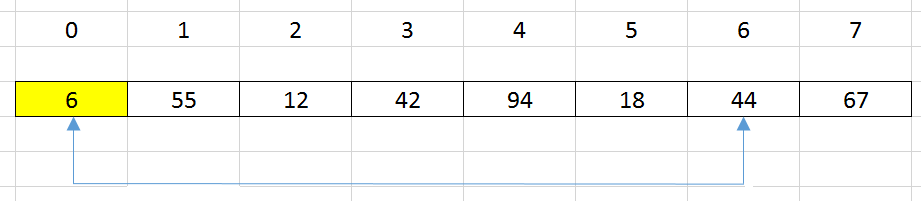3. At 1st position, select `a` as the minimum element of the array has range from 1 to `n - 1`.

• Iterate all elements from 2 to n - 1.

• Find the index = 2 is the minimum element.

• Swap `a` and `a`.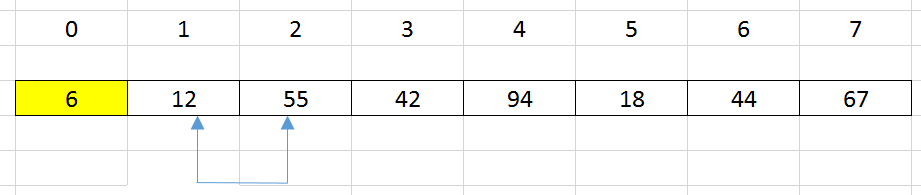Then, we have our array looks like that:4. At 2nd position, select `a` as the minimum element of the array has range from 2 to `n - 1`.

• Iterate all elements from 3 to n - 1.

• Find the index = 5 is the minimum element.

• Swap `a` and `a`.Then, we have our array looks like that: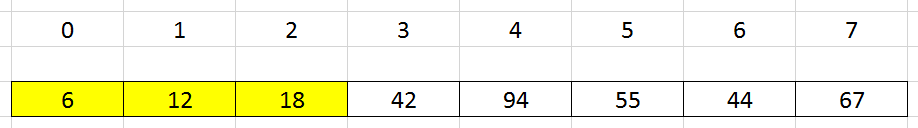5. At 3rd position, select `a` as the minimum element of the array has range from 3 to `n - 1`.

• Iterate all elements from 4 to n - 1.

• Find it is the minimum element, so we do not need to swap anything.

Then, we have our array looks like that: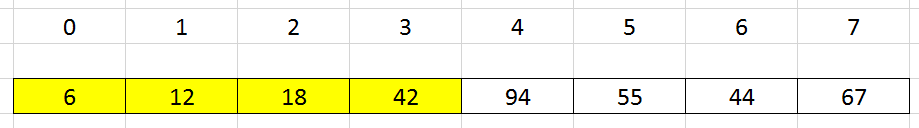6. At 4st position, select `a` as the minimum element of the array has range from 4 to `n - 1`.

• Iterate all elements from 5 to n - 1.

• Find the index = 6 is the minimum element.

• Swap `a` and `a`.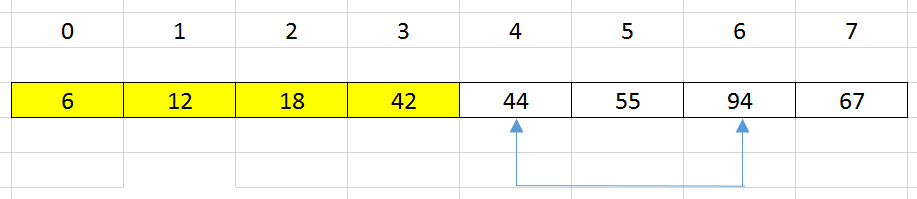Then, we have our array looks like that: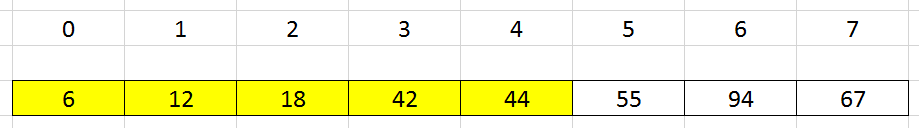7. Continue to do that, we have our final array.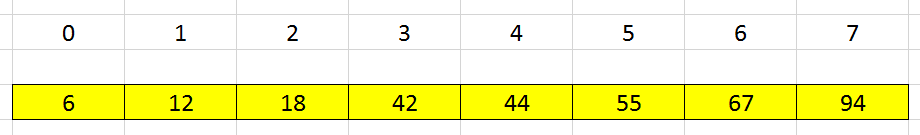## Source code

``````public static void selectionSort(int[] nums) {
int length = nums.length;

for (int i = 0; i < length; ++i) {
int minIndex = i;

for (int j = i + 1; j < length; ++j) {
if (nums[j] < nums[minIndex]) {
minIndex = j;
}
}

// swap value at the minIndex and i
int tmp = nums[minIndex];
nums[minIndex] = nums[i];
nums[i] = tmp;
}
}
``````

The complexity of the selection sort:

• Time complexity: O(n^2)
• Space complexity: O(1)

Selection sort is not stable sorting algorithm.

## When to use

• When our array has the small size.

• When our array is not partially sorted.

## Wrapping up

• Understanding the idea of the selection sort, and how to implement it.